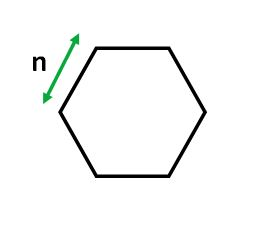GeeksforGeeks App
Open AppBrowser
Continue

A hexagon is a 6-sided, 2-dimensional geometric figure. The total of the internal angles of any hexagon is 720°. A regular hexagon has 6 rotational symmetries and 6 reflection symmetries. All internal angles are 120 degrees.Examples :

```Input: 4
Output: 41.5692

Input: 6
Output: 93.5307```

Number of vertices: 6
Number of edges: 6
Internal angle: 120°
Area = (3 √3(n)2 ) / 2

How does the formula work? There are mainly 6 equilateral triangles of side n and area of an equilateral triangle is (sqrt(3)/4) * n * n. Since in hexagon, there are total 6 equilateral triangles with side n, area of the hexagon becomes (3*sqrt(3)/2) * n * n

## C++

 `// CPP program to find ``// area of a Hexagon``#include ``#include ``using` `namespace` `std;`` ` `// function for calculating``// area of the hexagon.``double` `hexagonArea(``double` `s)``{``    ``return` `((3 * ``sqrt``(3) * ``            ``(s * s)) / 2);     ``}`` ` `// Driver Code``int` `main()``{``    ``// Length of a side ``    ``double` `s = 4; ``    ``cout << ``"Area : "``         ``<< hexagonArea(s);``    ``return` `0;``}`

## Java

 `import` `java.io.*;``public` `class` `GFG ``{ ``    ``// Create a function for calculating``    ``// the area of the hexagon.``    ``public` `static` `double` `hexagonArea(``double` `s) ``    ``{``        ``return` `((``3` `* Math.sqrt(``3``) * ``                ``(s * s)) / ``2``);``    ``} ``         ` `    ``// Driver Code``    ``public` `static` `void` `main(String[] args) ``    ``{     ``        ``// Length of a side``        ``double` `s = ``4``;      ``        ``System.out.print(``"Area: "` `+ ``                          ``hexagonArea(s) );``    ``}``}`

## Python3

 `# Python3 program to find``# area of a Hexagon``import` `math`` ` `# Function for calculating ``# area of the hexagon.``def` `hexagonArea(s):``     ` `    ``return` `((``3` `*` `math.sqrt(``3``) ``*` `            ``(s ``*` `s)) ``/` `2``); ``     ` `# Driver code     ``if` `__name__ ``=``=` `"__main__"` `: `` ` `    ``# length of a side. ``    ``s ``=` `4`` ` `    ``print``(``"Area:"``,``"{0:.4f}"` `. ``           ``format``(hexagonArea(s)))`` ` `# This code is contributed by Naman_Garg`

## C#

 `// C# program to find``// area of a Hexagon``using` `System;`` ` `class` `GFG ``{``     ` `    ``// Create a function for calculating``    ``// the area of the hexagon.``    ``public` `static` `double` `hexagonArea(``double` `s) ``    ``{``        ``return` `((3 * Math.Sqrt(3) * ``                ``(s * s)) / 2);``    ``} ``         ` `    ``// Driver Code``    ``public` `static` `void` `Main() ``    ``{``        ``// Length of a side ``        ``double` `s = 4; ``         ` `        ``Console.WriteLine(``"Area: "` `+ ``                           ``hexagonArea(s) );``    ``}``}`` ` `// This code is contributed by vt_m.`

## PHP

 ``

## Javascript

 ``

Output :

`Area: 41.5692`

Time Complexity: O(1)
Auxiliary Space: O(1), since no extra space has been taken.

My Personal Notes arrow_drop_up